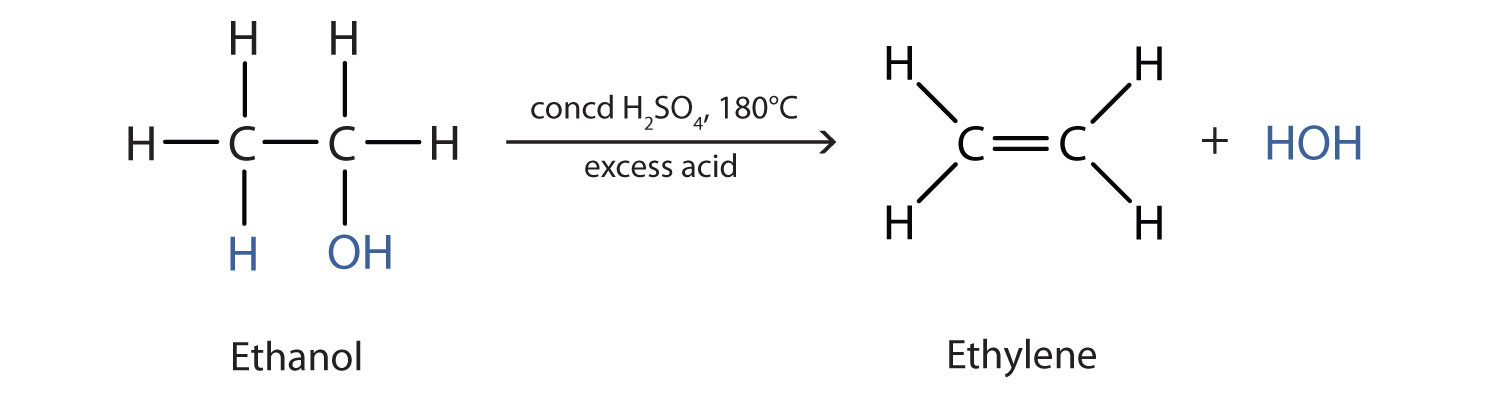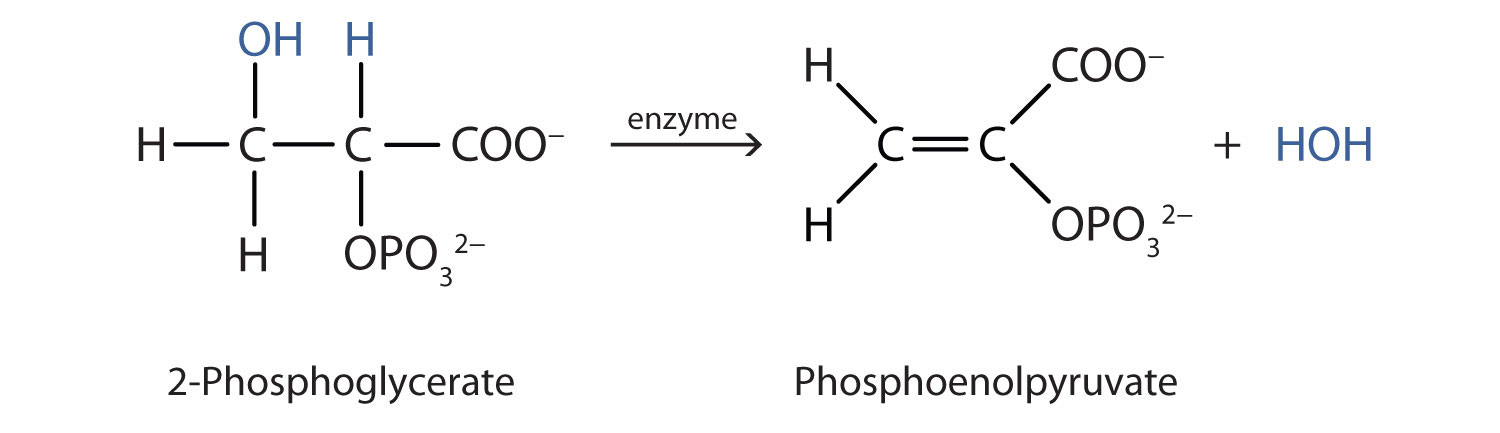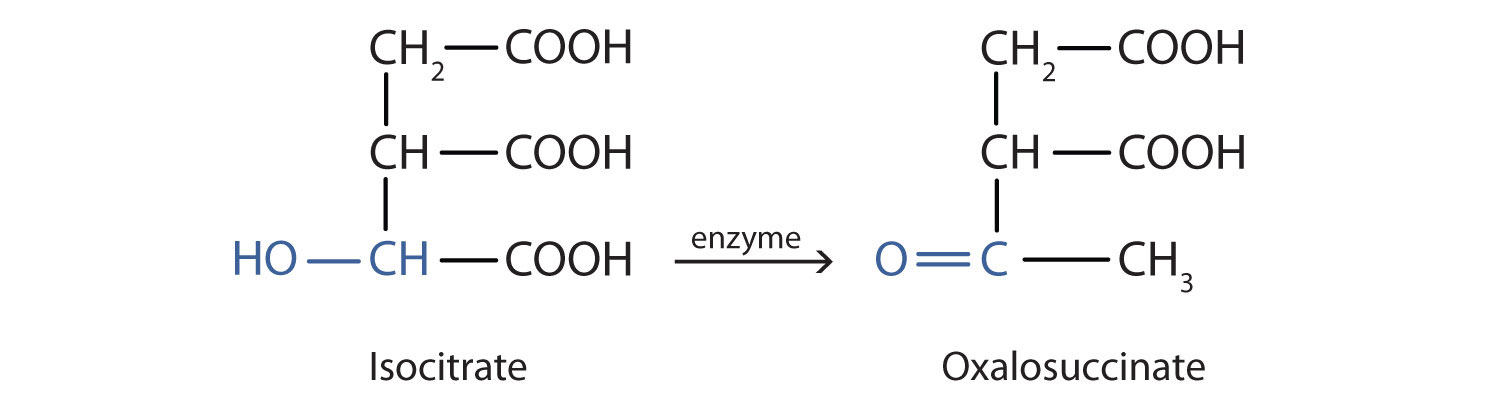Skip to main content

# 14.5: Reactions of Alcohols

Skills to Develop

1. Give two major types of reactions of alcohols.
2. Describe the result of the oxidation of a primary alcohol.
3. Describe the result of the oxidation of a secondary alcohol.

Chemical reactions in alcohols occur mainly at the functional group, but some involve hydrogen atoms attached to the OH-bearing carbon atom or to an adjacent carbon atom. Of the three major kinds of alcohol reactions, which are summarized in Figure $$\PageIndex{1}$$, two—dehydration and oxidation—are considered here. The third reaction type—esterification—is covered elsewhere.Figure $$\PageIndex{1}$$ Reactions of Alcohols. Oxidation and dehydration of alcohols are considered here.

## Dehydration

As noted in Figure $$\PageIndex{1}$$, an alcohol undergoes dehydration in the presence of a catalyst to form an alkene and water. The reaction removes the OH group from the alcohol carbon atom and a hydrogen atom from an adjacent carbon atom in the same molecule:Under the proper conditions, it is possible for the dehydration to occur between two alcohol molecules. The entire OH group of one molecule and only the hydrogen atom of the OH group of the second molecule are removed. The two ethyl groups attached to an oxygen atom form an ether molecule.(Ethers are discussed in elsewhere) Thus, depending on conditions, one can prepare either alkenes or ethers by the dehydration of alcohols.

Both dehydration and hydration reactions occur continuously in cellular metabolism, with enzymes serving as catalysts and at a temperature of about 37°C. The following reaction occurs in the "Embden–Meyerhof" pathwayAlthough the participating compounds are complex, the reaction is the same: elimination of water from the starting material. The idea is that if you know the chemistry of a particular functional group, you know the chemistry of hundreds of different compounds.

## Oxidation

Primary and secondary alcohols are readily oxidized. We saw earlier how methanol and ethanol are oxidized by liver enzymes to form aldehydes. Because a variety of oxidizing agents can bring about oxidation, we can indicate an oxidizing agent without specifying a particular one by writing an equation with the symbol [O] above the arrow. For example, we write the oxidation of ethanol—a primary alcohol—to form acetaldehyde—an aldehyde—as follows:We shall see that aldehydes are even more easily oxidized than alcohols and yield carboxylic acids. Secondary alcohols are oxidized to ketones. The oxidation of isopropyl alcohol by potassium dichromate (K2Cr2O7) gives acetone, the simplest ketone:Unlike aldehydes, ketones are relatively resistant to further oxidation, so no special precautions are required to isolate them as they form. Note that in oxidation of both primary (RCH2OH) and secondary (R2CHOH) alcohols, two hydrogen atoms are removed from the alcohol molecule, one from the OH group and other from the carbon atom that bears the OH group.

These reactions can also be carried out in the laboratory with chemical oxidizing agents. One such oxidizing agent is potassium dichromate. The balanced equation (showing only the species involved in the reaction) in this case is as follows:Alcohol oxidation is important in living organisms. Enzyme-controlled oxidation reactions provide the energy cells need to do useful work. One step in the metabolism of carbohydrates involves the oxidation of the secondary alcohol group in isocitric acid to a ketone group:The overall type of reaction is the same as that in the conversion of isopropyl alcohol to acetone.

Tertiary alcohols (R3COH) are resistant to oxidation because the carbon atom that carries the OH group does not have a hydrogen atom attached but is instead bonded to other carbon atoms. The oxidation reactions we have described involve the formation of a carbon-to-oxygen double bond. Thus, the carbon atom bearing the OH group must be able to release one of its attached atoms to form the double bond. The carbon-to-hydrogen bonding is easily broken under oxidative conditions, but carbon-to-carbon bonds are not. Therefore tertiary alcohols are not easily oxidized.

Example $$\PageIndex{1}$$

Write an equation for the oxidation of each alcohol. Use [O] above the arrow to indicate an oxidizing agent. If no reaction occurs, write “no reaction” after the arrow.

1. CH3CH2CH2CH2CH2OH
2.3.SOLUTION

The first step is to recognize the class of each alcohol as primary, secondary, or tertiary.

1. This alcohol has the OH group on a carbon atom that is attached to only one other carbon atom, so it is a primary alcohol. Oxidation forms first an aldehyde and further oxidation forms a carboxylic acid.2. This alcohol has the OH group on a carbon atom that is attached to three other carbon atoms, so it is a tertiary alcohol. No reaction occurs.3. This alcohol has the OH group on a carbon atom that is attached to two other carbon atoms, so it is a secondary alcohol; oxidation gives a ketone.Exercise $$\PageIndex{1}$$

Write an equation for the oxidation of each alcohol. Use [O] above the arrow to indicate an oxidizing agent. If no reaction occurs, write “no reaction” after the arrow.

1.2.3.## Summary

Alcohols can be dehydrated to form either alkenes (higher temperature, excess acid) or ethers (lower temperature, excess alcohol). Primary alcohols are oxidized to form aldehydes. Secondary alcohols are oxidized to form ketones. Tertiary alcohols are not readily oxidized.

## Concept Review Exercises

1. In a reaction, compound W with the molecular formula C4H10O is converted to compound X with the formula C4H8O. Is W oxidized, reduced, dehydrated, or none of these? Explain.

2. In a reaction, 2 mol of compound Y with the molecular formula C4H10O is converted to 1 mol of compound Z with the formula C8H18O. Is Y oxidized, reduced, or neither? Explain.

## Answers

1. oxidized; H is removed

2. neither; water is removed

## Exercises

1. Name the three major types of chemical reactions of alcohols.

2. Why do tertiary alcohols not undergo oxidation? Can a tertiary alcohol undergo dehydration?

3. Draw the structure of the product for each reaction.

1.2.4. Draw the structure of the product for each reaction.

1.2.5. Write an equation for the dehydration of 2-propanol to yield each compound type.

1. an alkene
2. an ether
6. Draw the structure of the alkene formed by the dehydration of cyclohexanol.

## Answers

1. dehydration, oxidation, and esterification

1.2.1. $$\mathrm{CH_3CHOHCH_3\underset{180^\circ C,\: excess\: acid}{\xrightarrow{conc\: H_2SO_4}} CH_3COCH_3+H_2O}$$
2. $$\mathrm{2CH_3CHOHCH_3 \underset{180^\circ C,\: excess\: acid}{\xrightarrow{conc\: H_2SO_4}}(CH_3)_2CHOCH(CH_3)_2+H_2O}$$